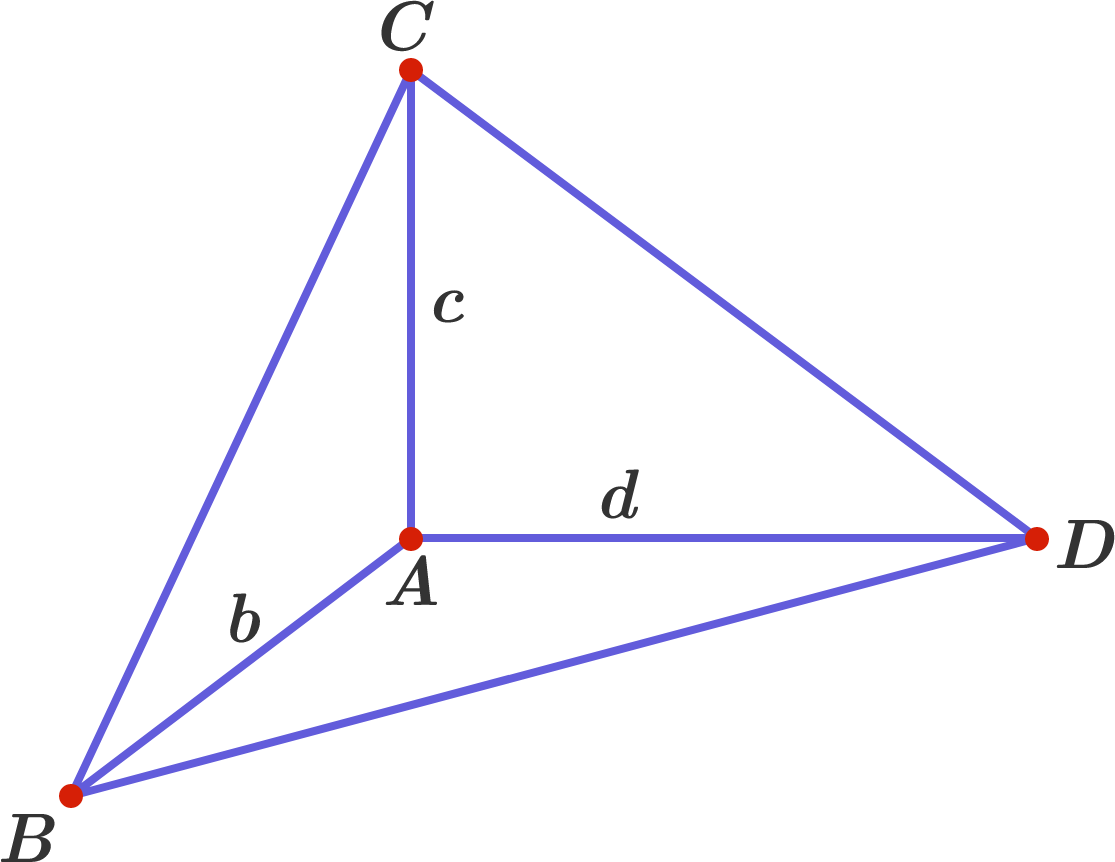# Integer Surface Area

$ABCD$ is a tetrahedron where all three face angles at vertex $A$ are right angles and the side lengths $AB = b, AC = c, AD = d$ are all distinct, positive integers.

What is the smallest possible integer value for the area of $\triangle BCD?$×